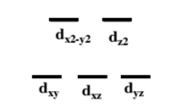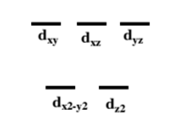# Problem: Draw the distribution of electrons for the central atoms in the following molecules using crystal field theoryK3[Cr(C2O4)3]•3H2O (octahedral)K2[Cu(C2O4)2]•2H2O (tetrahedral)

###### FREE Expert Solution

We're being asked to draw the distribution of electrons for the central atoms in the following molecules using crystal field theory.

Recall the energy splitting for each geometry.

For octahedral complexes:For tetrahedral complexes:For K3[Cr(C2O4)3]•3H2O (octahedral):

95% (385 ratings)###### Problem Details

Draw the distribution of electrons for the central atoms in the following molecules using crystal field theory

K3[Cr(C2O4)3]•3H2O (octahedral)

K2[Cu(C2O4)2]•2H2O (tetrahedral)

Frequently Asked Questions

What scientific concept do you need to know in order to solve this problem?

Our tutors have indicated that to solve this problem you will need to apply the Crystal Field Theory concept. If you need more Crystal Field Theory practice, you can also practice Crystal Field Theory practice problems.